GFG App
Open AppBrowser
Continue

# Class 9 NCERT Solutions- Chapter 13 Surface Areas And Volumes – Exercise 13.6

### Question 1. The circumference of the base of a cylindrical vessel is 132 cm and its height is 25 cm. How many liters of water can it hold? (1000 cm3 = 1l)

Solution: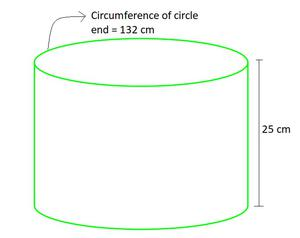Given values,

Circumference of the base of a cylindrical = 132 cm

Height of cylinder (h)= 25 cm

Base of cylinder is of circle shape, having circumference = 2πr (r is radius)

Hence, 2πr = 132 cm

r =(taking π=)

r =r = 21 cm

So, volume of cylinder = πr2h

= 22/7 × 21 × 21 × 25                                 (taking π=)

= 34650 cm3

As, 1000 cm3 = 1 litre

34650 cm3× 34650

== 34.650 litres

### Question 2. The inner diameter of a cylindrical wooden pipe is 24 cm and its outer diameter is 28 cm. The length of the pipe is 35 cm. Find the mass of the pipe, if 1 cm3 of wood has a mass of 0.6 g.

Solution: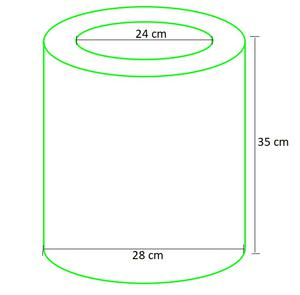Given values,

Inner radius of cylinder (r1)== 12 cm

Outer radius of cylinder (r2)== 14 cm

Height of cylinder (h)= 35 cm

So, volume used to make wood = volume of outer cylinder – volume of outer cylinder

= π(r22)h – π(r12)h

= π(r22 – r12)h× (142 – 122) × 35                     (taking π=)× (52) × 35

= 5720 cm3

As, 1 cm3 = 0.6 g

5720 cm3 = 0.6 × 5720 g

= 3432 grams

= 3.432 kg

### Which container has greater capacity and by how much?

Solution:

Let’s see each case,

(i) The shape of can is cuboid here, as having rectangular base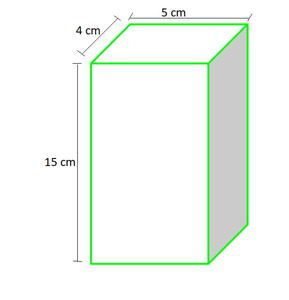Given values,

Length of can (l) = 5 cm

Width of can (b) = 4 cm

Height of can (h) = 15 cm

So, The amount of soft drink it can hold = volume of cuboid

= (l × b × h)

= 5 × 4 × 15 cm3

= 300 cm3

(ii)The shape of can is cylinder here, as having circular base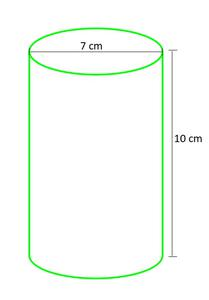Given values,

Radius of can (r) =cm

Height of can (h) = 10 cm

So, The amount of soft drink it can hold = volume of Cylinder

= (πr2h)× 10 cm3                                                  (taking π=)

= 385 cm3

Hence, we can see the can having circular base can contain (385 – 300 = 85 cm3) more amount of soft drinks than first can.

### Question 4. If the lateral surface of a cylinder is 94.2 cm2 and its height is 5 cm, then find

(ii) its volume. (Use π = 3.14)

Solution:

Given values,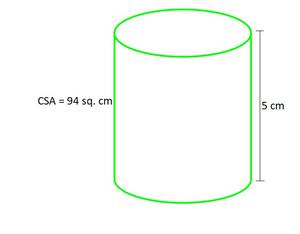Lateral surface of cylinder = 94.2 cm2

Height of cylinder (h) = 5 cm

Let’s see each case,

(i) So, the lateral surface is of rectangle shape whose

length = (circumference of base circle of cylinder) and width = height of cylinder

Let the base radius = r

Lateral surface = length × width

94.2 cm2 = (2πr) × h                         (circumference of circle = 2πr)

94.2 cm2 = (2 × 3.14 × r) × 5               (taking π = 3.14)

r =r = 3 cm

(ii) Given values,

Radius of cylinder (r)= 3 cm

So, the volume of cylinder = (πr2h)

= π × 3 × 3 × 5 cm3

= 3.14 × 3 × 3 × 5 cm3                    (taking π = 3.14)

= 141.3 cm3

### Question 5. It costs ₹2200 to paint the inner curved surface of a cylindrical vessel 10 m deep. If the cost of painting is at the rate of ₹20 per m2, find

(i) Inner curved surface area of the vessel,

(iii) Capacity of the vessel

Solution:

Given values,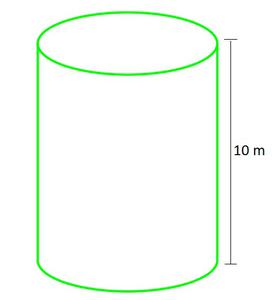Height of cylinder (h) = 10 m

Cost of painting rate = ₹20 per m2

Let’s see each case,

(i) For 1 m2 = ₹20

For lateral surface = ₹2200

So the lateral surface == 110 m2

(ii) Let the base radius = r

So as, Lateral surface = (circumference of base circle of cylinder) × height

110 m2= (2πr) × h

110 = (2 ×× r) × 10               (taking π=)

r =cm

r =cm

r = 1.75 cm

(iii) Volume of cylinder = (πr2h)× 10 cm3                  (taking π=)

= 96.25 cm3

### Question 6. The capacity of a closed cylindrical vessel of height 1 m is 15.4 liters. How many square meters of metal sheet would be needed to make it?

Solution:

Given values,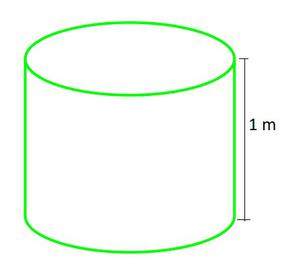Height of cylinder (h) = 1 m = 100 cm

Volume of cylinder (V) = 15.4 liters

As 1 liter = 1000 cm3

15.4 liters = 15.4 × 1000 cm3

V = 15,400 cm3

Volume of cylinder = (πr2h)

15,400 =× r2 × 100                                       (taking π=)

r2r2 = 49

r = √49

r = 7 cm

Surface area of a closed cylinder = (curve surface area + top and bottom circle) = 2πrh + (2 × πr2)

= 2πr (r+h)

= 2 ×× 7 × (7 + 100) cm2                                                   (taking π=)

= 2 × 22 × 107

= 4708 cm2

= 0.4708 m2

Hence, 0.4708 m2 of metal sheet would be needed to make it.

### Question 7. A lead pencil consists of a cylinder of wood with a solid cylinder of graphite filled in the interior. The diameter of the pencil is 7 mm and the diameter of the graphite is 1 mm. If the length of the pencil is 14 cm, find the volume of the wood and that of the graphite.

Solution:

So here pencil = (cylinder of wood + cylinder of graphite)

Given values,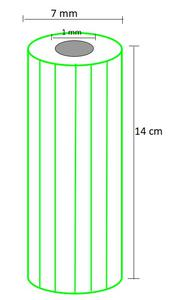Height of wood (and graphite) cylinder (h) = 14 cm = 140 mm

Radius of pencil (R)=mm

Radius of graphite (r)=mm

Volume of Graphite = (πr2h)× 140 mm3                                              (taking π=)

= 110 mm3

= 0.11 cm3

Volume of wood = Volume of pencil – Volume of graphite

= (πR2h) – (πr2h) = π(R2 – r2)h× (()2 – ()2) × 140 mm3                                      (taking π=)

= 22 × 20 × (–) mm3

= 22 × 20 × 12 mm3

= 5280 mm3

= 52.80cm3

### Question 8. A patient in a hospital is given soup daily in a cylindrical bowl of diameter 7 cm. If the bowl is filled with soup to a height of 4 cm, how much soup the hospital has to prepare daily to serve 250 patients?

Solution:

So here Volume of soup for each patient = Volume of cylinder.

Given values,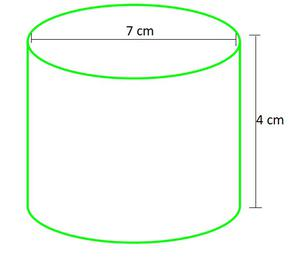Height of cylinder (h) = 4 cm

Radius of cylinder (r)=cm

Volume of Cylinder = (πr2h)×× 4 cm3                                             (taking π=)

= 154 cm

Volume of soup for 250 patient = 250 × Volume of cylinder.

= 250 × 154

= 38,500 cm3

Hence, 38,500cm3 soup is needed daily to serve 250 patients.

My Personal Notes arrow_drop_up